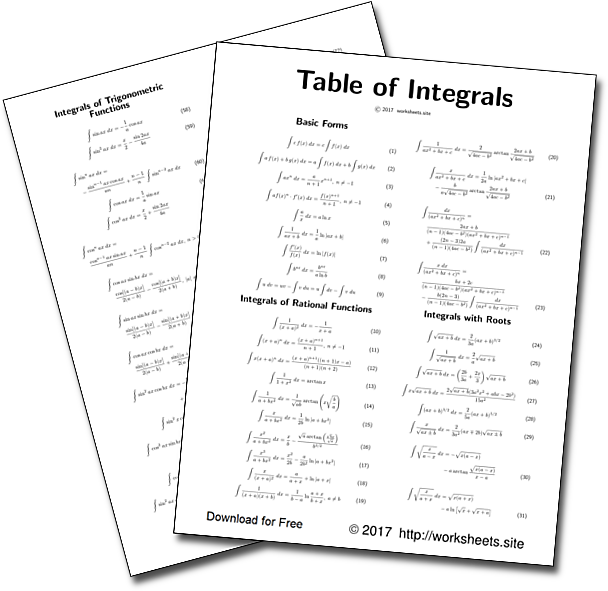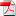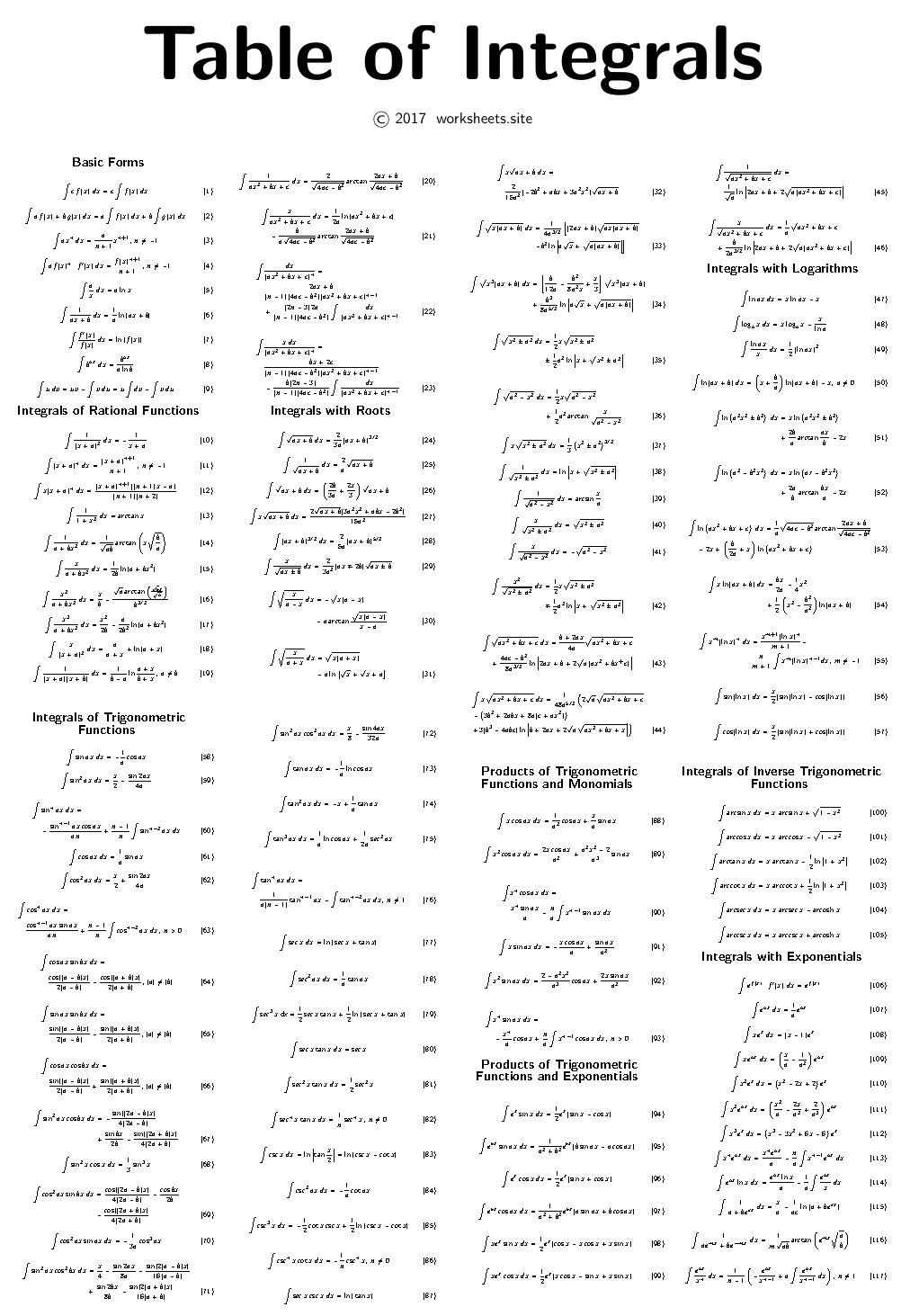# Printable Integrals Table

Complete table of integrals in a single sheet. Table of Trig Integrals. All the immediate integrals. Indefinite integrals. Free Table of Integrals to print on a single sheet side and side. Free Integration Worksheet. таблица интегралов.## 1. The Basics

Use the worksheets.site password to open the PDFfile. The PDF file has two pages to print on both sides of a single sheet. Once the sheet is printed side by side, it can be folded in half, to create a small booklet.This leaflet will help you solve calculus problems and make calculus homework easier. You can calculate standard indefinite integrals automatically, simply by looking for its pattern in the table and finding the primitive or antiderivative function. To save space we have omitted the integration constant. If the integral is definite then the table can be used to find the primitive and then you can evaluate it at the limits of integration.

The different methods by which the functions are transformed into more easily integrated ones can be classified as follows:

1. Integration by parts.
2. Partial Fractions.
3. Trigonometric Substitution.
4. Rationalization of numerators.
5. Combination with other integrals.
6. The substitution of a function of another variable with the independent variable of the integration.
7. Expansion of functions into infinite series.

## 2. Types of Integrals

The table presents a selection of integrals found in the Calculus books. It is a compilation of the most commonly used integrals. It includes:

1. Table of Basic Forms
2. Table of Rational Integrals
3. Table of Integrals with Roots
4. Table of Integrals with Logarithms
5. Table of Exponential Integrals
6. Table of Trigonometric Integrals
7. Table of Products of Trigonometric and Monomial Functions
8. Table of Products of Trigonometric and Exponential Functions
9. Table of Integrals of Reverse Trigonometric Functions

The first member of each equation contains the function to be integrated, the second member contains the expanded integral.

The entries in the table are generally ordered according to the integrand form. As in any dictionary, this arrangement makes it easier to locate them. But the ordering also matches surprisingly well with a grouping of different methods, since a special technique of integration is naturally associated with each category. Several reduction formulas are also included.